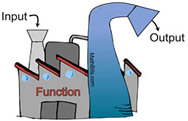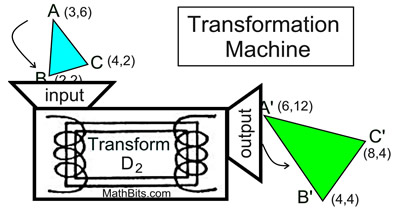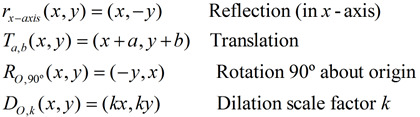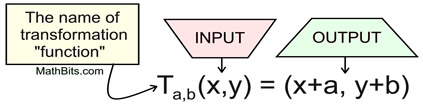Transformations as Functions MathBitsNotebook.com Terms of Use   Contact Person: Donna RobertsRemember those "function machines" from Algebra 1? You feed the machine INPUT and it spits out OUTPUT. For example, if you have a function defined as f (x) = 3x + 1, and you feed it x = 5, the function spits out f (5) = 16. Well, transformations can be viewed in this same manner.Transformations take points in the plane as inputs and give other points as outputs. As such, transformations behave like "functions".

As you have seen in your previous work with transformations, there are "rules" that define how a transformation takes "input" coordinates (from the pre-image) and creates "output" coordinates (for the image). These applied "rules" may result in translations, reflections, rotations, dilations, or a combination of changes to the original figure.

There are a variety of ways to write the "rules" that apply to transformations. The most common form for indicating transformation "rules" is a mapping notation, such as: (x, y) → (x + a, y + b).
The definitions of the classic transformations may appear in more of a functional notation form:Notice how this format resembles functional form: f (x) = 2x + 5 where x is the input and (2x + 5) is the output. More ways of indicating transformations appear in the section Rigid Transformations. Transformations can be viewed in terms of functions, where the inputs and outputs are points in the coordinate plane, rather than simply numerical values.